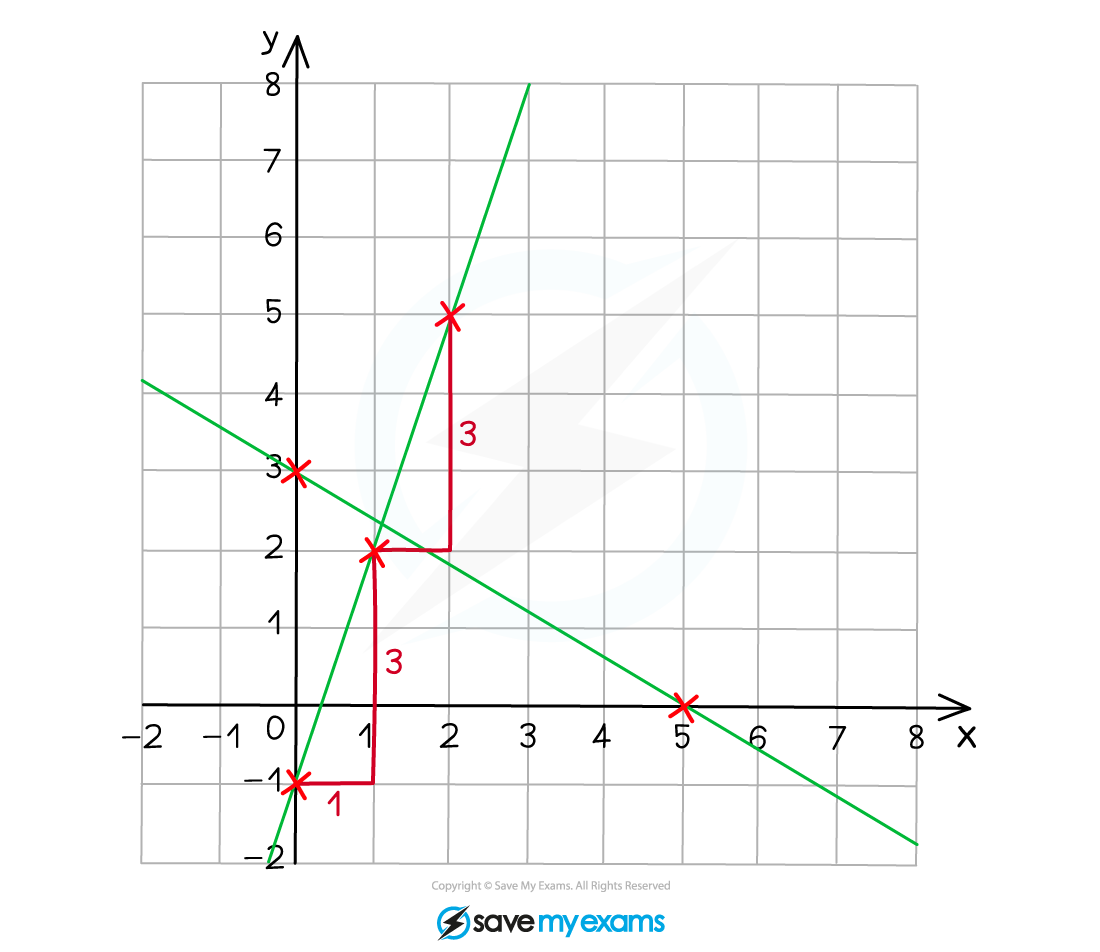# Edexcel IGCSE Maths 复习笔记 3.6.2 Straight Lines - Drawing Graphs

Edexcel IGCSE Maths 复习笔记 3.6.2 Straight Lines - Drawing Graphs

#### How do we draw the graph of a straight line from an equation?

• Before you start trying to draw a straight line, make sure you understand how to find the equation of a straight line – that will help you understand this
• How we draw a straight line depends on what form the equation is given in
• There are two main forms you might see:y = mx + c and ax + by = c
• Different ways of drawing the graph of a straight line:
1. From the form y = mx + c

(you might be able to rearrange to this form easily)plot c on the y-axis

go 1 across, m up (and repeat until you can draw the line)

2. From ax + by = c

put x = 0 to find y-axis interceptput y = 0 to find x-axis intercept

(You may prefer to rearrange to y = mx + c and use above method)

#### Exam Tip

It might be easier just to plot ANY two points on the line (a third one as a check is not a bad idea either) or use the TABLE function on your calculator.

#### Worked Example